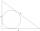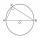# Isosceles III

The base of the isosceles triangle is 17 cm area 416 cm2. Calculate the perimeter of this triangle.

Result

p =  116.3 cm

#### Solution:Leave us a comment of example and its solution (i.e. if it is still somewhat unclear...):Be the first to comment!#### To solve this example are needed these knowledge from mathematics:

Pythagorean theorem is the base for the right triangle calculator. See also our trigonometric triangle calculator.

## Next similar examples:

1. ISO Triangle V2Perimeter of RR triangle (isosceles) is 474 m and the base is 48 m longer than the arms. Calculate the area of this triangle.
2. Isosceles triangleCalculate area and perimeter of an isosceles triangle ABC with base AB if a = 6 cm, c = 7 cm.
3. Isosceles trapezoidThe lengths of the bases of the isosceles trapezoid are in the ratio 5:3, the arms have a length of 5 cm and height = 4.8 cm. Calculate the circumference and area of a trapezoid.
4. Isosceles trapezoidCalculate the area of an isosceles trapezoid whose bases are in the ratio of 4:3; leg b = 13 cm and height = 12 cm.
5. ISO trapezoid v2bases of Isosceles trapezoid measured 12 cm and 5 cm and its perimeter is 21 cm. What is the are of a trapezoid?
6. RT 11Calculate the area of right tirangle if its perimeter is p = 45 m and one cathethus is 20 m long.
7. Triangle SAAThe triangle has one side long 71 m and its two internal angles is 60°. Calculate the perimeter and area of the triangle.
8. Circle inscribedCalculate the perimeter and area of a circle inscribed in a triangle measuring 3 , 4 and 5 cm.
9. SAS triangleThe triangle has two sides long 7 and 19 and included angle 36°. Calculate area of this triangle.
10. LandRectangular triangular land has area 30 square meters and 12 meters long leg. How many meters of the fence do you need for fencing this land?
11. Equilateral triangle v2Equilateral triangle has a perimeter 36 dm. What is its area?
12. MedianIn triangle ABC is given side a=10 cm and median ta= 13 cm and angle gamma 90°. Calculate length of the median tb.The double ladder is 8.5m long. It is built so that its lower ends are 3.5 meters apart. How high does the upper end of the ladder reach?The double ladder shoulders should be 3 meters long. What height will the upper top of the ladder reach if the lower ends are 1.8 meters apart?Determine the radius of the circumscribed circle to the right triangle with legs 9 cm and 6 cm.It is true that the middle traverse bisects the triangle?We want to prove the sentence: If the natural number n is divisible by six, then n is divisible by three. From what assumption we started?